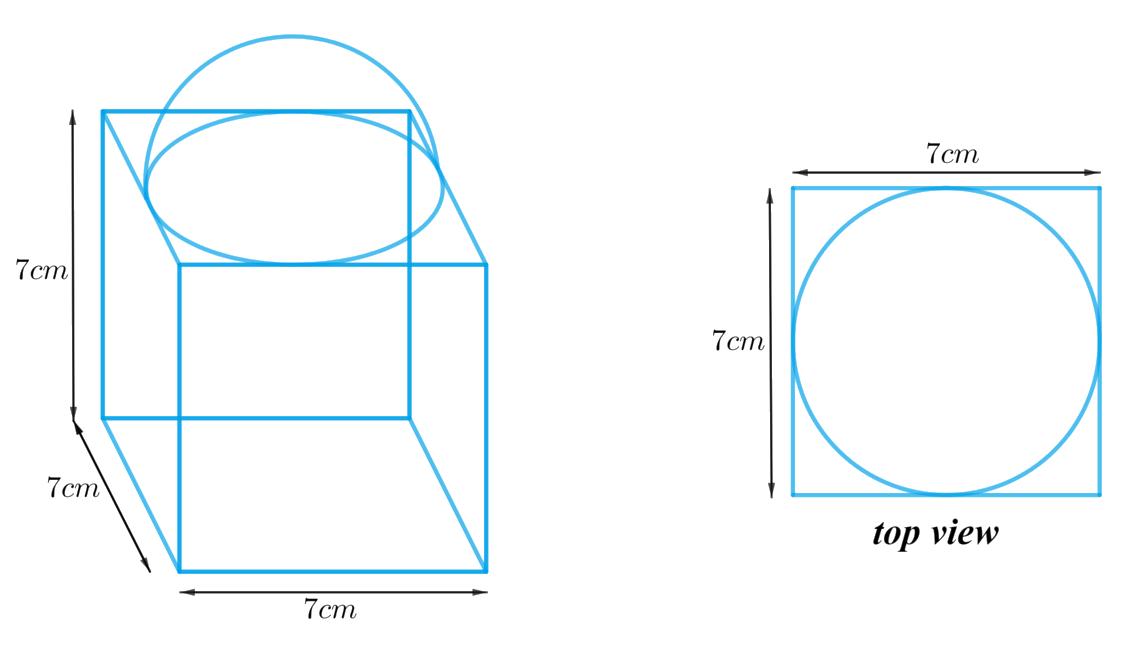# Ex.13.1 Q4 Surface Areas and Volumes Solution - NCERT Maths Class 10

Go back to  'Ex.13.1'

## Question

A cubical block of side $$7 \,\rm{cm}$$ is surmounted by a hemisphere. What is the greatest diameter the hemisphere can have? Find the surface area of the solid.

Video Solution
Surface Areas And Volumes
Ex 13.1 | Question 4

## Text Solution

What is known?

A cubical block of side $$7 \,\rm{cm}$$ is surmounted by a hemisphere.

What is unknown?

The greatest diameter the hemisphere can have and the surface area of the solid.

Reasoning:

We can create the figure of the solid as per given informationFrom the figure its clear that the greatest diameter the hemisphere can have is equal to the cube’s edge. otherwise a part of hemisphere’s rim (circumference of its circular base) will lie outside the top part of the cube.

As it’s clear from the top view of the figure that the top part of the cube is partially covered by hemispherical part.

Total surface area of the solid $$=$$ Surface Area of the cubical part $$-$$ Area of base of hemispherical part $$+$$ CSA of the hemispherical part

We will find the total area of the solid by using formulae;
CSA of the hemisphere $$= 2\pi {r^2}$$
Area of the base of the hemisphere $$= \pi {r^2}$$
where $$r$$ is the radius of the hemisphere

Surface area of the cube  $$= 6{l^2}$$
where $$l$$ is the length of the edge of the cube.

Steps:

Length of the edge of the cube,  $$l = 7 \rm cm$$

From the figure it’s clear that the greatest diameter the hemisphere can have is equal to the cube’s edge
Diameter of the hemisphere,  $$d = l = 7 \rm cm$$
Radius of the hemisphere, \begin{align}r = \frac{d}{2} = \frac{7}{2} \rm cm\end{align}

Total surface area of the solid $$=$$ Surface area of the cubical part $$–$$ Area of the base of the
hemispherical part $$+$$ CSA of the hemispherical part

\begin{align}&= 6{l^2} - \pi {r^2} + 2\pi {r^2}\\&= 6{l^2} + \pi {r^2}\\&= \!6 \!\times \! {\left( {7\rm {cm}} \right)^2} \!+ \! \frac{{22}}{7} \times \! {\left( {\frac{7}{2} \rm {cm}} \right)^2}\\&= 6 \times 49{ \rm c{m^2}} + \frac{{22}}{7} \times \frac{{49}}{4}{ \rm c{m^2}}\\&= 294{ \rm c{m^2}} + 38.5{ \rm c{m^2}}\\&= 332.5{ \rm c{m^2}}\end{align}

Learn from the best math teachers and top your exams

• Live one on one classroom and doubt clearing
• Practice worksheets in and after class for conceptual clarity
• Personalized curriculum to keep up with school